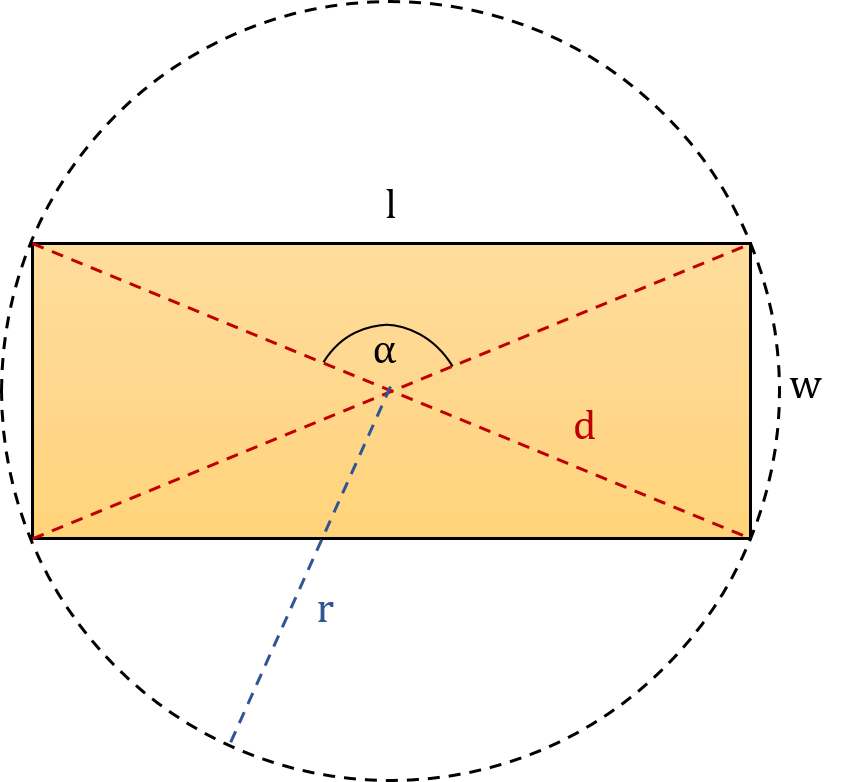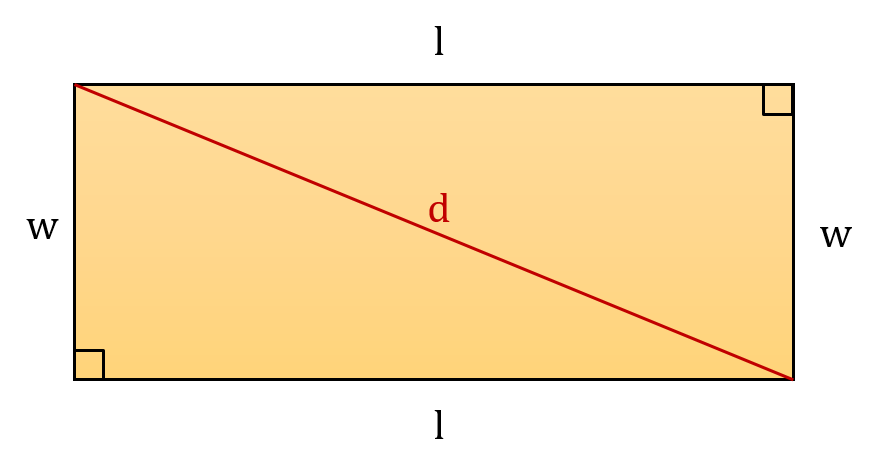# Diagonal of a Rectangle Calculator

Created by Dominik Czernia, PhD
Reviewed by Bogna Szyk
Last updated: Sep 21, 2023

Our diagonal of a rectangle calculator is an extensive tool that quickly finds the diagonal and other parameters of a rectangle. Have you met a specific rectangle problem and you don't know how to find the diagonal of a rectangle? Try entering a couple of parameters in the fields beside the text or keep reading to find out what are the possible diagonal of a rectangle formulas.

## Important parameters of rectangles

You can see a typical rectangle in the figure below. We have marked five basic quantities that describe a specific rectangle. You can use them to derive the diagonal of a rectangle formula. These are:• l – length;
• w – width;
• α – the angle between diagonals;
• d – diagonal;

and two other parameters which are not shown in the picture:

• A – area; and
• P – perimeter.

The term rectangle comes from the Latin rectangulus, which is a combination of two words: rectus (right, proper) and angulus (angle). This name derives from the fact that a rectangle is a quadrilateral with four right angles (4 × 90° = 360°). Its opposite sides are parallel and of equal length, and its two diagonals intersect each other in the middle and are of equal lengths too.

A square is a special case of a rectangle. Its definition is that it has all four sides of equal length; alternatively, the angle between two diagonals is right. Try our calculators dedicated to squares, e.g., the square calculator. They can quickly estimate the perimeter, area, and diagonal of every square you want, just from the length of its side.

The center of a rectangle is equidistant from its vertices; therefore, you can always circumscribe a circle on it. On the other hand, you can inscribe a circle into a rectangle only if it is a square.

## How do find the diagonal of a rectangle given sides?

To determine the diagonal of a rectangle, you need to:

1. Write down the sides of the rectangle, which we denote by w and l.
2. Square these two values. That is, compute l² and w².
3. Add together the two squared values from Step 2.
4. Take the square root of the result.
5. That's it! You've just found the length of the diagonal of your rectangle.

#### Why does this procedure work?

To derive the diagonal of a rectangle formula used above, divide a rectangle into two congruent right triangles, i.e., triangles with one angle of 90°. Each triangle will have sides of length l and w and a hypotenuse of length d. You can use the hypotenuse formula, e.g., from the Pythagorean theorem calculator, to estimate the diagonal of a rectangle, which can be expressed with the following formula:

d² = l² + w²

and now you should know how to find the diagonal of a rectangle explicit formula - just take a square root:

d = √(l² + w²)Our diagonal of a rectangle calculator allows you to use almost any units you want. Check out our length converter or area converter to find out how to switch between different units (SI and imperial).

## What's the diagonal of a rectangle formula?

Sometimes, you won't have given the length of two adjacent sides of a rectangle. How do you find the diagonal of a rectangle in that situation? The answer is provided with our diagonal of a rectangle calculator. First, let's write down three basic equations for the area, perimeter, and circumcircle radius:

1. Area of a rectangle: A = w × l,
2. Perimeter of a rectangle P = 2 × w + 2 × l,
3. Circumcircle radius of a rectangle r = d/2.

With the above equations, we can now derive various diagonal of a rectangle formulas that are used by this diagonal of a rectangle calculator:

1. Given length and width:
d = √(l² + w²);

2. Given length/width and area:
d = √(A²/l² + l²) or d = √(A²/w² + w²);

3. Given length/width and perimeter:
d = √(2l² - P × l + P²/4)) or d = √(2w² - P × w + P²/4);

4. Given length/width and angle:
d = w / sin(α/2) or d = l / cos(α/2);

5. Given area and perimeter:
d = ½√(P² - 8 × A);

6. Given area and angle:
d = √(2 × A / sin(α));

7. Given perimeter and angle:
d = P / (2 × sin(α/2) + 2 × cos(α/2)); and

d = 2 × r.

Note: The angle α between diagonals is in the front of the length like in the first figure. Also, remember that the diagonal of a rectangle calculator assumes that the length is longer than the width!

Did you know that there is a special rectangle called the golden rectangle? If not, head on to our golden rectangle calculator to learn how you can construct golden rectangles!

## FAQ

### What is the diagonal of a golden rectangle?

The answer is 1.176 times the longer side. If you know the shorter side of the golden rectangle, the diagonal's length is 1.902 times the length of the shorter side. To derive these answers, use the Pythagorean theorem.

### How do I calculate the diagonal of a rectangle given area and perimeter?

If you know the rectangle's area and perimeter, you can recover the diagonal using the formula:

diagonal = ½√(P² - 8 × A)

where P is the perimeter and A is the area of your rectangle.

### What is the diagonal of a rectangle with perimeter 20 and area 18?

The answer is 8. To get this answer, apply the formula diagonal = ½√(P² - 8 × A). Plugging in the data, we obtain diagonal = ½√(20² - 8 × 18) = ½√(400 - 144) = ½√256 = ½ × 16 = 8, as claimed.

Dominik Czernia, PhDLonger side (l)
in
Shorter side (w)
in
Area
in²
Perimeter
in
Angle between diagonals (α)
deg
in
Diagonal
Diagonal (d)
in
People also viewed…

### Body fat

Use the body fat calculator to estimate what percentage of your body weight comprises of body fat.

### Diamond problem

With our diamond problem calculator you'll solve any type of diamond problem.

### Millionaire

This millionaire calculator will help you determine how long it will take for you to reach a 7-figure saving or any financial goal you have. You can use this calculator even if you are just starting to save or even if you already have savings.

### Triangle slope

With the triangle slope calculator, you can find the slope of a line by drawing a triangle on it and determining the length of its sides.